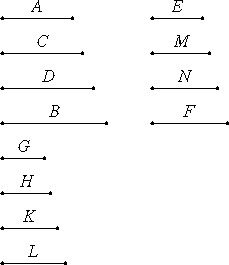# Proposition 8

If between two numbers there fall numbers in continued proportion with them, then, however many numbers fall between them in continued proportion, so many also fall in continued proportion between the numbers which have the same ratios with the original numbers.

Let the numbers C and D fall between the two numbers A and B in continued proportion with them, and make E in the same ratio to F as A is to B.

I say that, as many numbers as have fallen between A and B in continued proportion, so many also fall between E and F in continued proportion.As many as A, B, C, and D are in multitude, take so many numbers G, H, K, and L, the least of those which have the same ratio with A, C, D, and B. Then the extremes of them G and L are relatively prime.

VII.14

Now, since A, C, D, and B are in the same ratio with G, H, K, and L, and the multitude of the numbers A, C, D, and B equals the multitude of the numbers G, H, K, and L, therefore, ex aequali A is to B as G is to L. (V.11)

But A is to B as E is to F, therefore G is to L as E is to F.

But G and L are relatively prime, numbers which are relatively prime are also least, and the least numbers measure those which have the same ratio the same number of times, the greater the greater and the less the less, that is, the antecedent the antecedent and the consequent the consequent.

Therefore G measures E the same number of times as L measures F.

VII.Def.20

Next, let H and K measure M and N, respectively, as many times as G measures E. Then G, H, K, and L measure E, M, N, and F the same number of times. Therefore G, H, K, and L are in the same ratio with E, M, N, and F.

But G, H, K, and L are in the same ratio with A, C, D, and B, therefore A, C, D, and B are also in the same ratio with E, M, N, and F.

But A, C, D, and B are in continued proportion, therefore E, M, N, and F are also in continued proportion. Therefore, as many numbers as have fallen between A and B in continued proportion with them, so many numbers have also fallen between E and F in continued proportion.

Therefore, if between two numbers there fall numbers in continued proportion with them, then, however many numbers fall between them in continued proportion, so many also fall in continued proportion between the numbers which have the same ratios with the original numbers.

Q.E.D.

## Guide

The proposition says that if a : b = e : f and you can fit some number of terms between a and b in continued proportion (with a constant ratio), then you can fit the same number of terms between e and f in continued proportion.

For example, 18 : 50 = 63 : 175, with 18 : 30 : 50 a continued proportion, so one number can be fit between 63 and 175 in continued proportion. You can find it by reducing the original continued proportion to lowest terms 9 : 15 : 25, then scaling that up to the desired continued proportion 63 : 105 : 175.

#### Outline of the proof

Suppose that a : b = e : f and the sequence a, c, d, ..., b are in continued proportion. Use VII.33 to reduce that sequence to lowest terms g, h, k, ..., l. According to VIII.3, the ends of that sequence g and l are relatively prime. Since a : b = e : f, we also have g : l = e : f. But g is relatively prime to l, so the ratio g : l is in lowest terms (VII.21), and therefore g divides e the same number of times, say n, that l divides f (VII.20). Then the sequence ng, nh, nk, ..., nl is in continued proportion and starts with e and ends with f as required.

#### Implications

This proposition implies, among other things, that there is no number which forms a mean proportional between a number n and the number 2n, for if there were, there would be a number m so that 2, m, and 4 would form a continued proportion, but the only number between 2 and 4 is 3, and 2, 3, and 4 do not form a continued proportion. (If 1 is considered to be a number, the argument simplifies.)

We can translate this conclusion to modern terminology. The statement that there is no mean proportional between n and 2n says there is no number m such that n : m = m : 2n, and that says there is not rational number m/n whose square is 2. Thus, √2 is not a rational number.

See proposition X.9 for implications of this conclusion for incommensurability of line segments, that is, irrationality of surds like √2.

#### Use of this proposition

Although this proposition is not used in Book VIII, it is used in the first six propositions of Book IX.